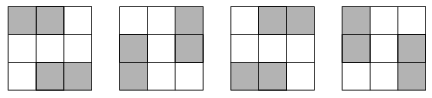Mathematical and Physical Journal
for High Schools
Issued by the MATFUND Foundation
 Already signed up? New to KöMaL?# Exercises and problems in InformaticsJanuary 2003

I. 40. Every row, column and both main diagonals of the square in the figure contain a prime number with five digits when rows and the diagonals are read from left to right, and columns from top to bottom. Moreover,

• the sum of the digits of the prime numbers must be the same,

• the digit in the top left corner is given in advance,

• any prime number can be re-used in the actual square and

• a prime number can not have any zero leading digit, that is, for example, 00003 is not a prime number with five digits.

 1 1 3 5 1 3 3 2 0 3 3 0 3 2 3 1 4 0 3 3 3 3 3 1 1

Your program (I40.pas, ...) should read the sum of the digits of the prime numbers, further the digit to be placed into the top left corner, then display the corresponding square.

In the example, the sum of the digits=11, while the top left digit=1.

(10 points)

I. 41. A square is divided into smaller light or dark squares. We can obtain different figures by rotating the original square or reflecting it around its axes of symmetry. (However, it may happen that some transformations, or combination of transformations produce the same - possibly the original - figure.)

Write your program (I41.pas, ...) which reads the colouring of the NxN square using the symbols V (=light) and S (=dark), successively representing in each row the colours of the small squares, then displays the square together with all different images under all combinations of transformations.

The example shows for N=3 the original square=((S,S,V),(V,V,V),(V,S,S)) and all possible images under the transformations.(10 points)

I. 42. The exchange rates between 100 units of some foreign currency and HUF (=Ft) are stored in a table. For example, 100 AUD is worth 13279 HUF.

Prepare a sheet (I42.xls) which - using a fixed table of foreign currency - computes all the exchange rates in the fourth column if any amount of a single (but arbitrary) currency in the third column has been entered.

See the examples.

 100 HUF amount value AUD 13279 176,96 CAD 15014 156,51 CHF 16287 144,28 CZK 777 3024,32 EUR 23835 98,59 HUF 100 23499,00 PLZ 6051 388,35 SEK 2618 897,59 SKK 580 4051,55 USD 23499 100 100,00

 100 HUF amount value AUD 13279 2,93 CAD 15014 2,59 CHF 16287 2,39 CZK 777 50 50,00 EUR 23835 1,63 HUF 100 388,50 PLZ 6051 6,42 SEK 2618 14,84 SKK 580 66,98 USD 23499 1,65

(10 points)